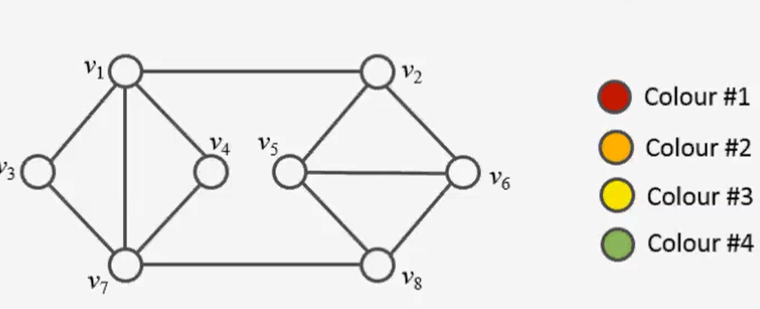## Vertex Coloring using ILPIn this post I’ll show you how to solve the vertex coloring problem to optimality using linear programming. Since the problem is NP-complete, there is no algorithm which can solve any problem instance in deterministic polynomial time. In a former post, I used constraint programming to find an optimal (minimal) vertex coloring. A popular greedy… Read more Vertex Coloring using ILP

## Graph Coloring ProblemA node coloring is valid or admissible if any two adjacent nodes do not have the same color. If a graph is colorable, there is a smallest number such that the graph is node colorable. This number is called the chromatic number of the graph and is usually denoted by . The problem to identify… Read more Graph Coloring Problem